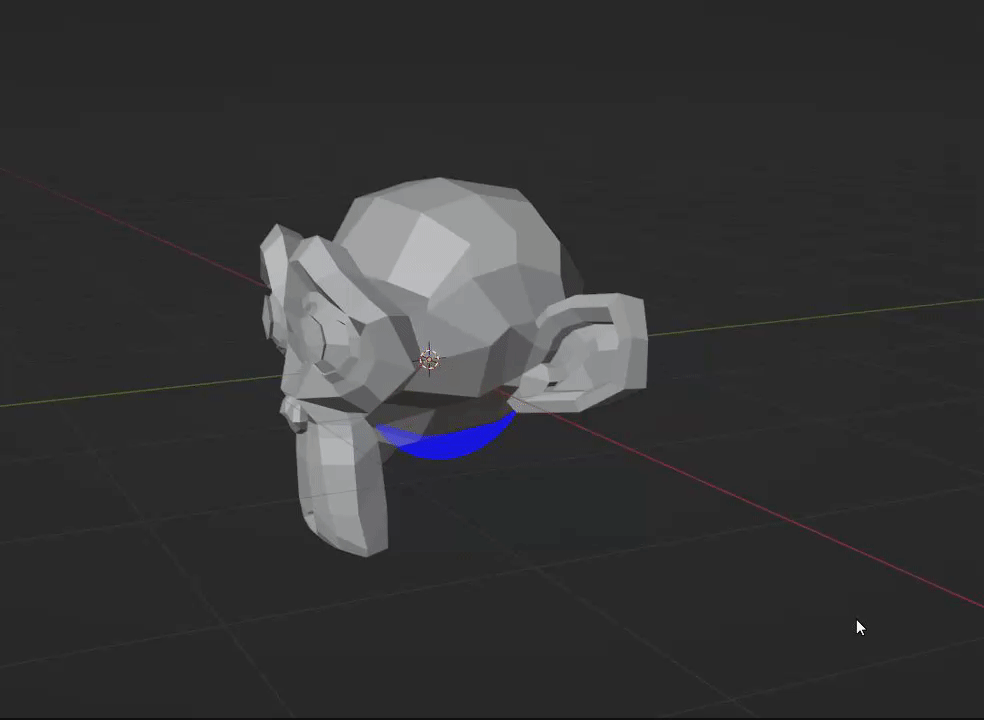# Gizmo culled depth doesn't take effect when mouse is hovered on it

I have created a `GizmoGroup` with the option `DEPTH_3D` enabled, which, according to the docs, “Supports culled depth by other objects in the view.” This works great until I move my mouse cursor over the Gizmo which causes it to appear in front of all objects. This only happens if I have draw_select defined. However, it seems that I need draw_select defined to listen for click events. Is this an intentional feature? Is there any way to disable this behavior?

Here is what I mean:To reproduce what I have, just run this script in an empty blend file. It will create all the necessary objects.

``````import bpy
import math
from mathutils import Vector
from typing import Optional

class DiscGizmo(bpy.types.Gizmo):
bl_idname = "OBJECT_GT_bound_disc"

def __init__(self):
super().__init__()

def draw(self, context):
self.draw_select(context, None)

def draw_select(self, context, select_id):
return

self.color = 0, 0, 1
self.alpha = 0.5
self.color_highlight = self.color
self.alpha_highlight = self.alpha

self.draw_custom_shape(

def generate_mesh(self, obj: bpy.types.Object):
"""Generate ``self.mesh`` and ``self.wireframe_mesh``"""

height = 0.5
segments = 32

tris = self.generate_tris(verts)

self.mesh = self.new_custom_shape("TRIS", tris)

@staticmethod
def generate_verts(radius: float, height: float, segments: int = 16):
vertices: list[Vector] = []

for i in range(segments):
angle = (2 * math.pi) * (i / segments)

# Oriented on the y-z plane

top_vertex = Vector((height / 2, y, z))
bottom_vertex = Vector((-height / 2, y, z))

vertices.append(top_vertex)
vertices.append(bottom_vertex)

return vertices

@staticmethod
def generate_tris(vertices: list[Vector]):
# Two triangles per face
num_tris = len(vertices)
tris: list[Vector] = []

# Side faces
for i in range(num_tris):
for j in range(3):
vert_ind = i + j

# Wrap back around to beginning of verts list if end is reached
if i + j >= len(vertices):
vert_ind = (i + j) % len(vertices)

tris.append(vertices[vert_ind])

# Top faces
for i in range(num_tris):
if i + 2 < len(vertices):
neighbor = vertices[i + 2]
else:
neighbor = vertices[(i + 2) % len(vertices)]

tris.append(vertices[i])
tris.append(neighbor)
tris.append(Vector((vertices[i].x, 0, 0)))

return tris

class BoundGizmoGroup(bpy.types.GizmoGroup):
bl_idname = "OBJECT_GGT_Bounds"
bl_label = "Bound Shapes"
bl_space_type = "VIEW_3D"
bl_region_type = "WINDOW"
bl_options = {"3D", "PERSISTENT", "DEPTH_3D"}

def setup(self, context):
for obj in context.view_layer.objects:
if obj.hide_get():
continue

if obj.name == "bound_disc":
gz = self.gizmos.new(DiscGizmo.bl_idname)
gz.use_draw_modal = True
gz.generate_mesh(obj)

bpy.utils.register_class(BoundGizmoGroup)
bpy.utils.register_class(DiscGizmo)

if "bound_disc" not in bpy.data.objects:
obj = bpy.data.objects.new("bound_disc", object_data=None)
obj.empty_display_size = 0.0001

if "Suzanne" not in bpy.data.objects:
``````

Some context if interested:

I’m trying to create Gizmos that represent collision volumes for a import/exporter. In the addon, every object has a bound type property that the GizmoGroup will check for. Depending on the bound type, it will create a Gizmo representing that primitive collision volume based off some properties defined in the addon (i.e obj.bound_radius, obj.bound_height, etc). I excluded that stuff though to produce a more minimal example.

Looks like depth is not fully supported atm. From the source code, it appears that culling is disabled when the object is highlighted:

``````/* Weak! since we don't 100% support depth yet (select ignores depth)
* always show highlighted. */
if (is_depth && (gz->state & WM_GIZMO_STATE_HIGHLIGHT)) {
is_depth = false;
}
``````

Hopefully this will be changed soon.